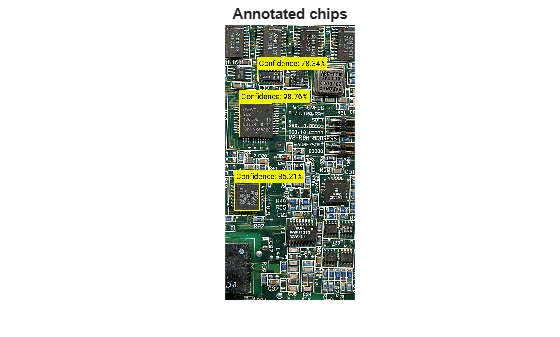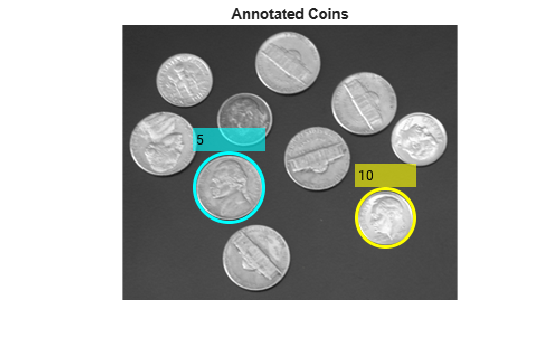# insertObjectAnnotation

Annotate truecolor or grayscale image or video stream

## Syntax

``RGB = insertObjectAnnotation(I,shape,position,label)``
``RGB = insertObjectAnnotation(I,shape,position,label,Name,Value)``
``insertObjectAnnotation(I,'rectangle',position,label)``
``insertObjectAnnotation(I,'circle',position,label)``

## Description

example

````RGB = insertObjectAnnotation(I,shape,position,label)` returns a truecolor image annotated with `shape` and `label` at the location specified by `position`.```

example

````RGB = insertObjectAnnotation(I,shape,position,label,Name,Value)` uses additional options specified by one or more `Name,Value` pair arguments.```

example

````insertObjectAnnotation(I,'rectangle',position,label)` inserts rectangles and labels at the location indicated by the position matrix.```
````insertObjectAnnotation(I,'circle',position,label)` inserts circles and corresponding labels at the location indicated by the position matrix.```

## Examples

collapse all

`I = imread('board.tif');`

Create labels of floating point numbers. The floating point numbers relate to confidence value labels.

```label_str = cell(3,1); conf_val = [85.212 98.76 78.342]; for ii=1:3 label_str{ii} = ['Confidence: ' num2str(conf_val(ii),'%0.2f') '%']; end```

Set the position for the rectangles as [x y width height].

` position = [23 373 60 66;35 185 77 81;77 107 59 26];`

Insert the labels.

```RGB = insertObjectAnnotation(I,'rectangle',position,label_str,... 'TextBoxOpacity',0.9,'FontSize',18);```

Display the annotated image.

```figure imshow(RGB) title('Annotated chips');````I = imread('coins.png');`

Set positions for the circles. The first two values represents the center at (x,y) and the third value is the radius.

`position = [96 146 31;236 173 26];`

Set the label to display the integers 5 and 10 (U.S. cents).

`label = [5 10];`

Insert the annotations.

`RGB = insertObjectAnnotation(I,'circle',position,label,'LineWidth',3,'Color',{'cyan','yellow'},'TextColor','black');`

Display.

```figure imshow(RGB) title('Annotated coins');```## Input Arguments

collapse all

Truecolor or grayscale image, specified as an image or video stream. The input image can be either an M-by-N-by-3 truecolor or a M-by-N 2-D grayscale image.

Data Types: `double` | `single` | `uint8` | `uint16` | `int16`

Rectangle or circle annotation, specified as a character vector indicating the annotation shape.

Data Types: `char`

Location and size of the annotation shape, specified as an M-by-3 or M-by-4 matrix. When you specify a rectangle, the position input matrix must be an M-by-4 matrix. Each row, M, specifies a rectangle as a four-element vector, [x y width height]. The elements, x and y, indicate the upper-left corner of the rectangle, and the width and height specify the size.

When you specify a circle, the position input matrix must be an M-by-3 matrix, where each row, M, specifies a three-element vector [x y r]. The elements, x and y, indicate the center of the circle and r specifies the radius.

Example: `position = [50 120 75 75]`

A rectangle with top-left corner located at x=50, y=120, with a width and height of 75 pixels.

Example: `position = [96 146 31]`

A circle with center located at x=96, y=146 and a radius of 31 pixels.

Example: ```position = [23 373 60 66;35 185 77 81;77 107 59 26]```

Location and size for three rectangles.

Label to associate with a shape, specified as an M numeric vector, string, categorical labels, or a cell array of ASCII character vectors. The character, string, or categorical labels must be encoded as ASCII characters. The cell array must be the of length equal to the number of shape positions. You can specify a scalar label for all shapes as a numeric scalar, string, or categorical.

Example: label = [5 10], where the function marks the first shape with the label, 5, and the second shape with the label, 10.

### Name-Value Pair Arguments

Specify optional comma-separated pairs of `Name,Value` arguments. `Name` is the argument name and `Value` is the corresponding value. `Name` must appear inside quotes. You can specify several name and value pair arguments in any order as `Name1,Value1,...,NameN,ValueN`.

Example: `'Color'`, `'white'` sets the color for the label text box to white.

Font face of text, specified as the comma-separated pair consisting of '`Font`' and a character vector. The font face must be one of the available truetype fonts installed on your system. To get a list of available fonts on your system, type `listTrueTypeFonts` at the MATLAB® command prompt.

Data Types: `char`

Label text font size, specified as the comma-separated pair consisting of `'FontSize'` and an integer corresponding to points in the range of [8 72].

Data Types: `double` | `single` | `int8` | `int16` | `int32` | `int64` | `uint8` | `uint16` | `uint32` | `uint64`

Shape border line width, specified as the comma-separated pair consisting of '`LineWidth`' and a positive scalar integer in pixels.

Color for shape and for corresponding label text box, specified as the comma-separated pair consisting of `'Color'` and either a character vector, an [R G B] vector, a cell array, or an M-by-3 matrix.

To specify one color for all shapes, set this parameter to either a character vector or an [R G B] vector. To specify a color for each of the M shapes, set this parameter to a cell array of M character vectors. Alternatively, you can specify an M-by-3 matrix of RGB values for each annotation. RGB values must be in the range of the input image data type.

Supported colors: `'blue'`, `'green'`, `'cyan'`, `'red'`, `'magenta'`, `'black'`, and `'white'`.

Data Types: `char` | `uint8` | `uint16` | `int16` | `double` | `single` | `cell`

Color of text in text label, specified as the comma-separated pair consisting of `'TextColor'` and either a character vector, an [R G B] vector, a cell array, or an M-by-3 matrix. To specify one color for all text, set this parameter to either a character vector or an [R G B] vector. To specify a color for each of the M text labels, set this parameter to a cell array of M character vectors. Alternatively, you can specify an M-by-3 matrix of RGB values for each annotation. RGB values must be in the range of the input image data type.

Supported colors: `'blue'`, `'green'`, `'cyan'`, `'red'`, `'magenta'`, `'yellow'`, and `'white'`.

Data Types: `char` | `uint8` | `uint16` | `int16` | `double` | `single` | `cell`

Opacity of text label box background, specified as the comma-separated pair consisting of `'TextBoxOpacity'` and a scalar defining the opacity of the background of the label text box. Specify this value in the range of `0` to `1`.

Data Types: `double` | `single` | `int8` | `int16` | `int32` | `int64` | `uint8` | `uint16` | `uint32` | `uint64`

## Output Arguments

collapse all

Truecolor image with annotation, returned as an image or video stream.

Data Types: `double` | `single` | `uint8` | `uint16` | `int16`